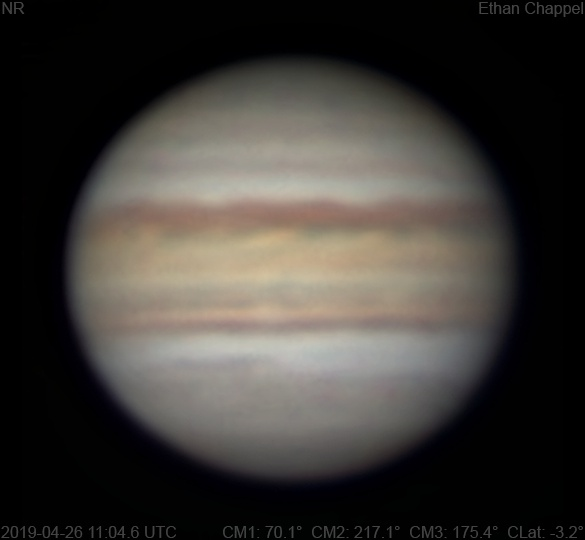# Jupiter 2019-04-26 11:04 UTC

CM1: 70.10°

CM2: 217.10°

CM3: 175.30°

CLat: -3.20°

Description

This imaging session was quite frustrating to get started. I intended to quickly align the telescope with the Moon, but could not find it with the telescope at all! When I finally aligned the scope and aimed at Jupiter, seeing looked very good. However, a group of clouds blocked the view right as I was going to start capturing. When they finally moved away, the seeing had become awful. I also forgot to switch filters on one capture, causing me more frustration with the threat of more clouds looming.

Notes:

• The Equatorial Zone is orange.
• There is a bright spot in the South Equatorial Belt, north of Oval BA.
• Oval BA is near the central meridian.

Equipment

ZWO ASI174MM

Celestron X-Cel 3x

Celestron EdgeHD 8

Logs
```FireCapture v2.6  Settings
------------------------------------
Observer=Ethan Chappel
Camera=ZWO ASI174MM
Filter=B
Profile=Saturn
Diameter=16.99"
Magnitude=0.46
CMI=142.7° CMIII=92.0°  (during mid of capture)
FocalLength=9600mm (F/34)
Resolution=0.13"
Filename=2019-04-26-1108_4-EC-B-Sat.ser
Date=2019_04_26
Start=11_07_10.551
Mid=11_08_25.557
End=11_09_40.564
Start(UT)=11_07_10.551
Mid(UT)=11_08_25.557
End(UT)=11_09_40.564
Duration=150.013s
Date_format=yyyy_MM_dd
Time_format=HH_mm_ss
LT=UT -6h
Frames captured=11256
File type=SER
Binning=no
Bit depth=8bit
Debayer=no
ROI=452x444
ROI(Offset)=0x0
FPS (avg.)=75
Shutter=13.28ms
Gain=400 (100%)
AutoExposure=off
Brightness=1 (off)
Gamma=50 (off)
HighSpeed=off
SoftwareGain=10 (off)
USBTraffic=80 (off)
AutoGain=off
AutoHisto=75 (off)
FPS=100 (off)
Histogramm(min)=0
Histogramm(max)=146
Histogramm=57%
Noise(avg.deviation)=2.66
AutoAlign=false
PreFilter=none
Limit=150 Seconds
Sensor temperature=30.7°C
FireCapture v2.6  Settings
------------------------------------
Observer=Ethan Chappel
Camera=ZWO ASI174MM
Filter=G
Profile=Saturn
Diameter=16.99"
Magnitude=0.46
CMI=140.6° CMIII=90.0°  (during mid of capture)
FocalLength=9600mm (F/34)
Resolution=0.13"
Filename=2019-04-26-1104_8-EC-G-Sat.ser
Date=2019_04_26
Start=11_03_35.043
Mid=11_04_50.047
End=11_06_05.052
Start(UT)=11_03_35.043
Mid(UT)=11_04_50.047
End(UT)=11_06_05.052
Duration=150.009s
Date_format=yyyy_MM_dd
Time_format=HH_mm_ss
LT=UT -6h
Frames captured=11900
File type=SER
Binning=no
Bit depth=8bit
Debayer=no
ROI=452x444
ROI(Offset)=0x0
FPS (avg.)=79
Shutter=12.56ms
Gain=400 (100%)
AutoExposure=off
Brightness=1 (off)
Gamma=50 (off)
HighSpeed=off
SoftwareGain=10 (off)
USBTraffic=80 (off)
AutoGain=off
AutoHisto=75 (off)
FPS=100 (off)
Histogramm(min)=0
Histogramm(max)=152
Histogramm=59%
Noise(avg.deviation)=2.70
AutoAlign=false
PreFilter=none
Limit=150 Seconds
Sensor temperature=30.5°C
FireCapture v2.6  Settings
------------------------------------
Observer=Ethan Chappel
Camera=ZWO ASI174MM
Filter=R
Profile=Saturn
Diameter=16.99"
Magnitude=0.46
CMI=138.1° CMIII=87.6°  (during mid of capture)
FocalLength=9900mm (F/35)
Resolution=0.12"
Filename=2019-04-26-1100_5-EC-G-Sat.ser
Date=2019_04_26
Start=10_59_19.972
Mid=11_00_34.977
End=11_01_49.982
Start(UT)=10_59_19.972
Mid(UT)=11_00_34.977
End(UT)=11_01_49.982
Duration=150.010s
Date_format=yyyy_MM_dd
Time_format=HH_mm_ss
LT=UT -6h
Frames captured=7851
File type=SER
Binning=no
Bit depth=8bit
Debayer=no
ROI=452x444
ROI(Offset)=0x0
FPS (avg.)=52
Shutter=19.07ms
Gain=400 (100%)
AutoExposure=off
Brightness=1 (off)
Gamma=50 (off)
HighSpeed=off
SoftwareGain=10 (off)
USBTraffic=80 (off)
AutoGain=off
AutoHisto=75 (off)
FPS=100 (off)
Histogramm(min)=0
Histogramm(max)=168
Histogramm=65%
Noise(avg.deviation)=2.68
AutoAlign=false
PreFilter=none
Limit=150 Seconds
Sensor temperature=30.1°C
```